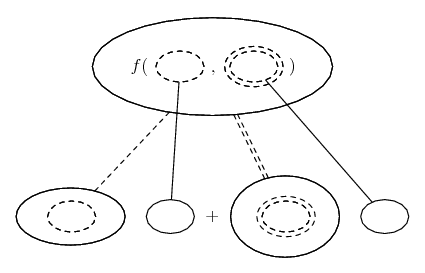# Thread: First order exact equations

1. ## First order exact equations

I have a problem that has eaten hours of my time. Each time I work it I get a different answer, which suggests it's an issue with algebra. Since I'm dysgraphic it's highly likely that I'm dropping negatives or making arithmetic errors.Anyway, I need a step by step solution on this problem. Your solution will go into my notes on this section.

3(X^2)(Y^-2) + (X^-2)(Y^2) - (2X^-3) + ((6Y^-4) - 2(X^3)(Y^-3) - (2X^-1)(Y))Y' = 0, Y(1)=-1

This will need an integrating factor since M_x = 6XY^-2 -2X^-3Y^2 and N_y = -24Y^-5 + 6X^3Y^-4 -2X^-1 thus M_x is not equal to N_y. This is where things start going to heck for me!

2. ## Re: First order exact equationsOriginally Posted by WolvenmoonThis will need an integrating factor since M_x = 6XY^-2 -2X^-3Y^2 and N_y = -24Y^-5 + 6X^3Y^-4 -2X^-1 thus M_x is not equal to N_y.
Happily, no, it is exact. Just in case a picture helps...... where (key in spoiler) ...

Spoiler:is the chain rule for two inner functions, i.e...

\frac{d}{dx}\ f(u(x), v(x)) = \frac{\partial f}{\partial u} \frac{du}{dx} + \frac{\partial f}{\partial v} \frac{dv}{dx}

As with...... the ordinary chain rule, straight continuous lines differentiate downwards (integrate up) with respect to x, and the straight dashed line similarly but with respect to the (corresponding) dashed balloon expression which is (one of) the inner function(s) of the composite expression.

Full size: here

More here: Exact equations

_________________________________________

Don't integrate - balloontegrate!

Balloon Calculus; standard integrals, derivatives and methods

Balloon Calculus Drawing with LaTeX and Asymptote!

#### Search Tags

equations, exact, order